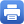Columbia Home
Modern Geometry

This course is taken in sequence, part 1 in the fall, and part 2 in the spring.

### MODERN GEOMETRY I

I Differential Manifolds

• Smooth manifolds
• Smooth maps (submersions, immersions, embeddings)
• Tangent spaces and tangent bundles
• Vector bundles
• Vector fields (flows, Frobenius theorem)
• Lie groups and homogeneous spaces
• Covering maps and fibrations
• Tensors, differential forms, Stokes’ theorem

II Riemannian Geometry

• Riemannian metrics and connections
• Metrics and connections on vector bundles
• Geodesics
• Curvature
• Jacobi fields
• Isometric immersions (second fundamental form)

### MODERN GEOMETRY II

I Riemannian Geometry (continued)

• Complete manifolds
• Manifolds of constant curvature
• First and second variations of energy
• Bonnet-Myers theorem, Synge’s theorem

II Principal Bundles

• Principal bundles and associated bundles
• Connections and curvatures on principal bundles
• Induced connections and curvatures on associated vector bundles
• Parallel transport and holonomy
• Characteristic classes

III Witten’s proof of the Positive Energy TheoremPrint this page